# Construct Binary Tree from Inorder and PostOrder Traversal

In this article, we will solve the most asked coding interview problem: Construct A Binary Tree from Inorder and PostOrder Traversal.

Given Inorder and PostOrder Traversal of a binary tree, we need to construct the unique binary tree represented by them.

```Example: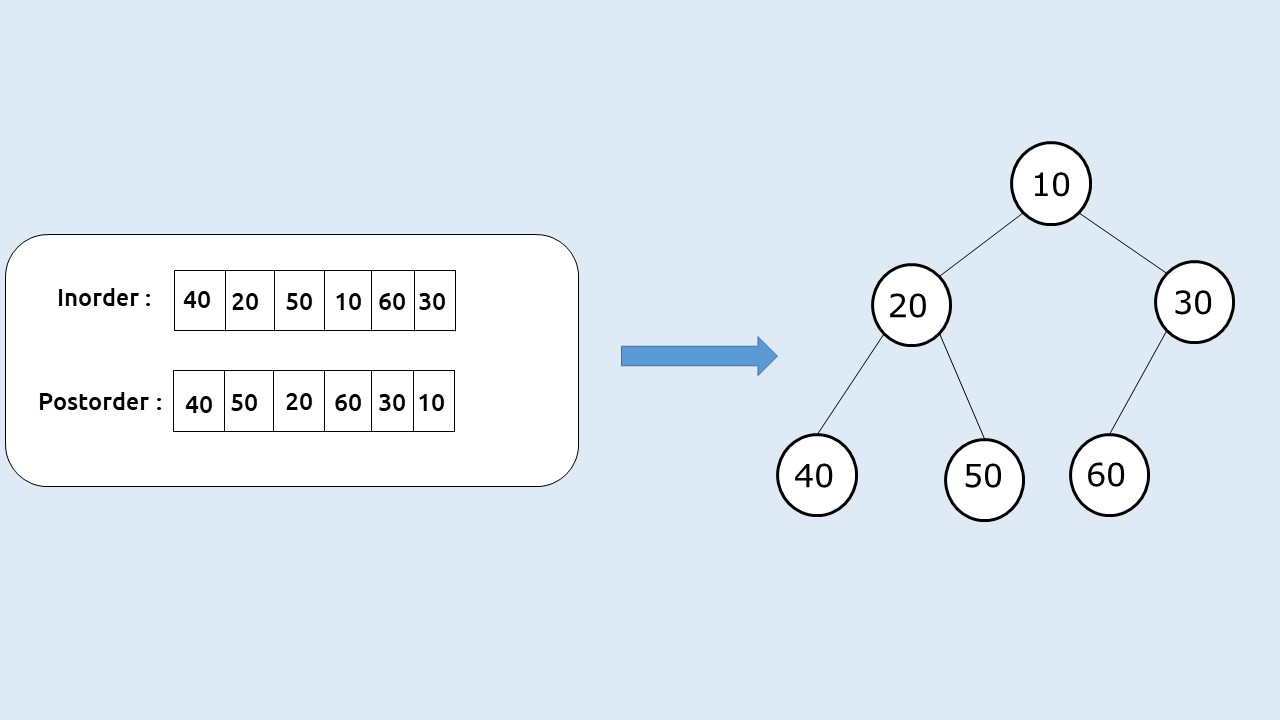```

Disclaimer: Don’t jump directly to the solution, try it out yourself first.

Solution:

In this article, we learned that a unique binary tree can be constructed using a postorder and an inorder traversal. Here we will discuss the solution.

Intuition:

Inorder traversal is a special traversal that helps us to identify a node and its left and right subtree. Postorder traversal always gives us the root node as its last element. Using these properties we can construct the unique binary tree.

Given this example: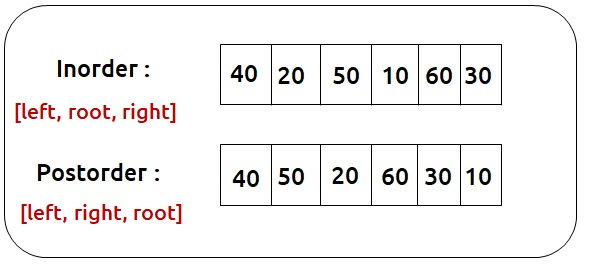Here 10 (last element of postorder) is the root element. So we can find its index in the inorder traversal(say elem). The left subtree of the root will be present to the left side of inorder whereas the right subtree of root will be present on the right side of elem in the inorder traversal:

We can define a recursive function that creates one node at a time. First, we create the root node, and then we can take the help of recursion to create its left and right subtrees. In order to make recursion work, we need to provide the correct inorder and postorder traversal of the subtree for every recursive call.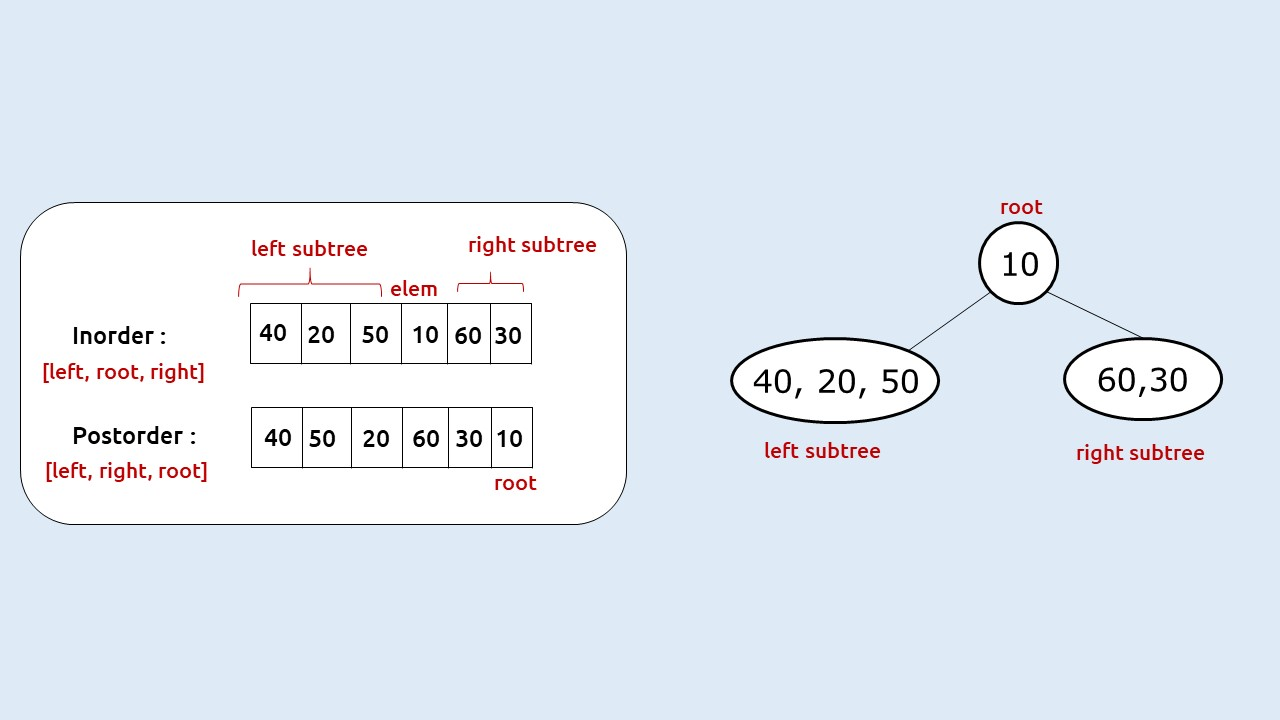To make more efficient function calls we can use variables (inStart, inEnd) and (postStart and postEnd) in order to point to the start and end of the inorder and postorder traversal respectively, and avoid copying of arrays.

Next, we need to figure out how we are going to search the root index in the inorder traversal. For this, we have two options: Linear Search and Hashmaps. We will choose the second one because it will return us the index in constant time. Before making the first recursive call, we will simply add all the (value, index) pairs to a map and pass it to our recursive function.

If n is the size of the Inorder traversal/Postorder traversal. Then our first function call will be :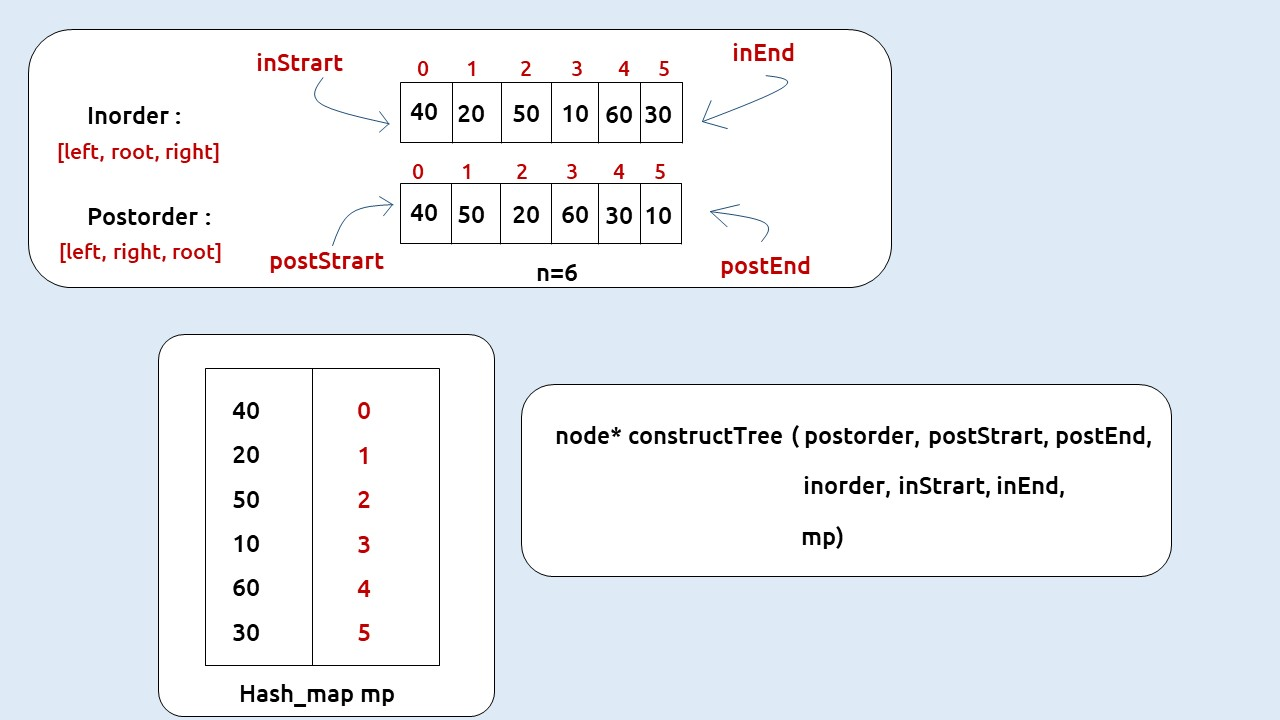Now the main task left is to pass the correct postStart, postEnd, inStart, inEnd to the respective recursive calls for the left and right subtree. We can calculate the number of elements in the left subtree from the root index, say nElems (elem – InStart, where elem is the index of root in inorder traversal). As inorder is [left, root, right] and postorder is [left, right, root] the number of elements (nElems) will easily tell us the preorder and inorder traversal of the subtrees according to the following table: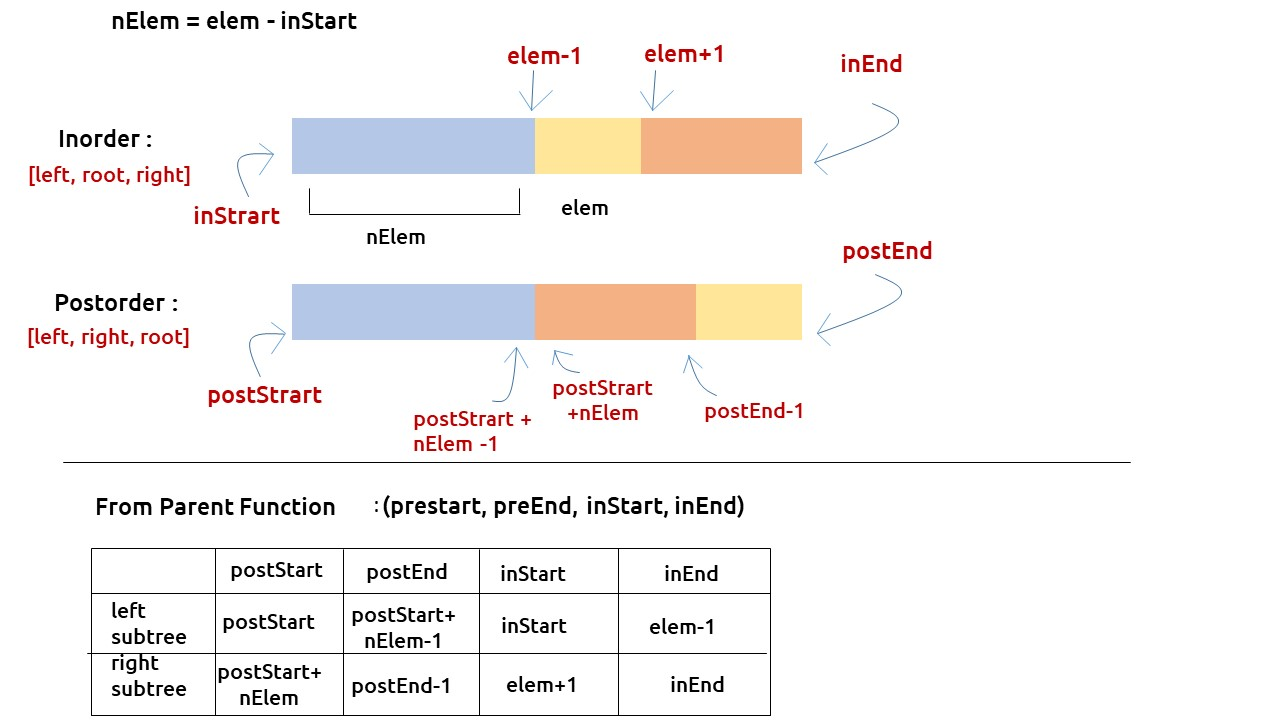The base case will be when inStart> inEnd or postStart > postEnd, in that case, we can simply return NULL.

Approach:

The algorithm approach can be stated as:

• Create a map to store the inorder indexes.
• Call the function constructTree with all 7 parameters as shown above.
• In the recursive function, first check the base case, if (postStart,>postEnd || inStart> inEnd) then return NULL.
• Construct a node (say root) with the root value( last element of postorder).
• Find the index of the root, say elem from the hashmap.
• Find the number of elements ( say nElem) in the left subtree  = elem – inStart
•  Call recursively for the left subtree with correct values (shown in the above table) and store the answer received in root->left.
• Call recursively for the right subtree with correct values (shown in the above table) and store the answer received in root->right.
• Return root

Dry Run: To understand a detailed dry run of this approach, please watch the video attached below.

Code:

## C++ Code

``````#include <bits/stdc++.h>

using namespace std;

struct node {
int data;
struct node * left, * right;
};

struct node * newNode(int data) {
struct node * node = (struct node * ) malloc(sizeof(struct node));
node -> data = data;
node -> left = NULL;
node -> right = NULL;

return (node);
}
node * constructTree(vector < int > & postorder, int postStart, int postEnd,
vector < int > & inorder, int inStart, int inEnd, map < int, int > & mp) {
if (postStart > postEnd || inStart > inEnd) return NULL;

node * root = newNode(postorder[postEnd]);
int elem = mp[root -> data];
int nElem = elem - inStart;

root -> left = constructTree(postorder, postStart, postStart + nElem - 1,
inorder, inStart, elem - 1, mp);
root -> right = constructTree(postorder, postStart + nElem, postEnd-1, inorder,
elem + 1, inEnd, mp);

return root;
}

node * buildTree(vector < int > & postorder, vector < int > & inorder) {
int postStart = 0, postEnd = postorder.size() - 1;
int inStart = 0, inEnd = inorder.size() - 1;

map < int, int > mp;
for (int i = inStart; i <= inEnd; i++) {
mp[inorder[i]] = i;
}

return constructTree(postorder, postStart, postEnd, inorder, inStart, inEnd, mp);
}

int main() {

vector<int> postorder{40,50,20,60,30,10};
vector<int> inorder{40,20,50,10,60,30};
node * root = buildTree(postorder, inorder);
return 0;
}
``````

Time Complexity: O(N)

Assumption: Hashmap returns the answer in constant time.

Space Complexity: O(N)

Reason: We are using an external hashmap of size ‘N’.

## Java Code

``````class Solution {
public TreeNode buildTree(int[] inorder, int[] postorder) {
if (inorder == null || postorder == null || inorder.length !=
postorder.length)
return null;
HashMap<Integer, Integer> hm = new HashMap<Integer,Integer>();
for (int i=0;i<inorder.length;++i)
hm.put(inorder[i], i);
return buildTreePostIn(inorder, 0, inorder.length-1, postorder, 0,
postorder.length-1,hm);
}

private TreeNode buildTreePostIn(int[] inorder, int is, int ie, int[] postorder,
int ps, int pe,HashMap<Integer,Integer> hm){
if (ps>pe || is>ie) return null;
TreeNode root = new TreeNode(postorder[pe]);
int ri = hm.get(postorder[pe]);
TreeNode leftchild = buildTreePostIn(inorder, is, ri-1, postorder, ps,
ps+ri-is-1, hm);
TreeNode rightchild = buildTreePostIn(inorder,ri+1, ie, postorder,
ps+ri-is, pe-1, hm);
root.left = leftchild;
root.right = rightchild;
return root;
}
}``````

Time Complexity: O(N)

Assumption: Hashmap returns the answer in constant time.

Space Complexity: O(N)

Reason: We are using an external hashmap of size ‘N’.

Special thanks to Anshuman Sharma for contributing to this article on takeUforward. If you also wish to share your knowledge with the takeUforward fam, please check out this article